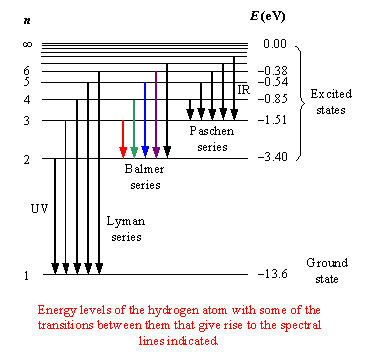A# hydrogen spectrumThe hydrogen spectrum is a characteristic pattern of spectral lines, either absorption or emission, produced by the hydrogen atom. The various series of lines are named according to the lowest energy level involved in the transitions that give rise to the lines.

The Lyman series involve jumps to or from the ground state (n=1); the Balmer series (in which all the lines are in the visible region) corresponds to n=2, the Paschen series to n = 3, the Brackett series to n = 4, and the Pfund series to n = 5.

To illustrate with specific values, consider the Balmer series, in which the four principal lines, designated as H-alpha, H-beta, H-gamma, and H-delta, involve energy jumps of 3.02 × 10-19, 4.07 × 10-19, 4.57 × 10-19, and 4.84 × -19 joules (J), respectively, and corresponding photons of wavelengths are 656.3, 486.1, 434.0, and 410.2 nanometers (nm).